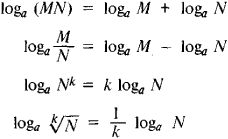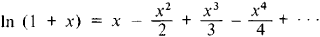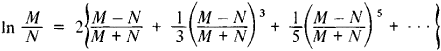# logarithm

(redirected from Napier logarithm)
Also found in: Dictionary, Thesaurus, Medical.

## logarithm

(lŏg`ərĭthəm) [Gr.,=relation number], number associated with a positive number, being the power to which a third number, called the base, must be raised in order to obtain the given positive number. For example, the logarithm of 100 to the base 10 is 2, written log10 100=2, since 102=100. Logarithms of positive numbers using the number 10 as the base are called common logarithms; those using the number e (see separate article) as the base are called natural logarithms or Napierian logarithms (for John NapierNapier, John
, 1550–1617, Scottish mathematician and theologian. He invented logarithms and wrote Mirifici logarithmorum canonis descriptio (1614), containing the first logarithmic table and the first use of the word logarithm.
). The natural logarithm of a number x is denoted by ln x or simply log x. Since logarithms are exponentsexponent,
in mathematics, a number, letter, or algebraic expression written above and to the right of another number, letter, or expression called the base. In the expressions x2 and xn, the number 2 and the letter n
, they satisfy all the usual rules of exponents. Consequently, tedious calculations such as multiplications and divisions can be replaced by the simpler processes of adding or subtracting the corresponding logarithms. Logarithmic tables are generally used for this purpose.

## Logarithm

The logarithm of a number N to the base a is the exponent m to which a (base of the logarithm) must be raised in order to obtain N (denoted by logaN). Thus m = logaN if am = N. For example, log10 100 = 2, log2 (1/32) = –5, and loga 1 = 0 since 100 = 102, 1/32 = 2–5, and1 = a0. For negative a infinitely many positive numbers would not have real logarithms, and therefore it is assumed that a > 0 and a ≠ 1. It follows from the properties of the logarithmic function that a unique real logarithm corresponds to every positive number (logarithms of negative numbers are complex numbers).

The basic properties of logarithms areThese properties enable us to reduce multiplication and division of numbers to the addition and subtraction of their logarithms, raising a number to a power to multiplication of its logarithm by the exponent, and extraction of a root of a number to division of its logarithm by the index of the root, that is, to simpler operations.

When the base a is fixed, one speaks of a particular logarithmic system. Common (Briggs’) logarithms (a = 10), denoted by log N, are the most frequently used logarithms, owing to the decimal nature of our counting system. Common logarithms of rational numbers different from 10k with integral k are transcendental numbers that can be approximately expressed as decimal fractions. The integral part of a common logarithm is called the characteristic, and the fractional part the mantissa. Since log (10kN) = K + log N, the common logarithms of numbers that differ by a factor of 10k have the same mantissa and differ only in their characteristic. This property is the basis for constructing tables of logarithms containing only the mantissa of the logarithms of integers.

Natural (Napierian) logarithms (denoted by In N), whose base is the transcendental number e = 2.71828 . . ., are also of great importance. Change of base of logarithms can be accomplished using the formula logb N = loga N/loga b. The relations connecting natural and common logarithms are

In N = log N/ log e

log N = In N/1n 10

1/log e = 2.30258

1/ln 10 = 0.43429. . .

History. The discovery of logarithms was connected with the rapid development of astronomy in the 16th century, the refinement of astronomical observations, and the increasing complexity of astronomical computations. The authors of the first logarithmic tables proceeded from the existing dependence between the properties of a geometric progression and those of the arithmetic progression constructed from the exponents of its terms. These relationships, which had already been observed by Archimedes (third century B.C.), were well known to N. Chuquet (1484) and to the German mathematician N. Stiefel (1544). The first logarithmic tables were compiled about the same time but independently by J. Napier (1614, 1619) and the Swiss mathematician J. Bürgi (1620).

An important step in the theoretical study of logarithms was made by the Belgian mathematician Gregory of St. Vincent (1647), who discovered the relationship between logarithms and areas bounded by an arc of a hyperbola and the axis of abscissas and the corresponding ordinates. A representation of logarithms by means of an infinite power series was provided by N. Mercator (1668), who found thatShortly thereafter J. Gregory (1668) discovered the expansionThis series converges very rapidly if M = N + 1 and if N is sufficiently large and therefore can be used for computing logarithms. Of great importance in the development of the theory of logarithms was the work of L. Euler, who established the idea that taking a logarithm is the inverse of the operation of raising to a power.

The term “logarithm,” proposed by J. Napier, arises from combining the Greek words logos (here, “ratio”) and arithmos (“number”). In ancient mathematics, the square, cube, and higher powers of a/b were called binary, ternary, and higher ratios of a/b. Thus, for Napier the words logu arithmos denoted the “number (multiplicity) of the ratio”; that is, Napier understood the logarithm as an auxiliary number for measuring the ratio of two numbers. The term “natural logarithm” is due to N. Mercator, and the term “characteristic” to the British mathematician H. Briggs. The terms “mantissa” and “base” of a logarithm, in our sense, are due to L. Euler. The modern definition of logarithm was first given by the British mathematician W. Gardiner in 1742. The logarithm symbol is derived from the abbreviation of “logarithm” and is encountered in different forms almost simultaneously with the appearance of the first tables: for example, J. Kepler used “Log” (1624) as did Briggs (1631), while “log” and “1” were used by B. Cavalieri (1632, 1643).

### REFERENCES

Markushevich, A. I. Ploshchadi i logarifmy. Moscow-Leningrad, 1952. Istorila matematiki, vol. 2. Moscow, 1970.

## logarithm

[′läg·ə‚rith·əm]
(mathematics)
The real-valued function log u defined by log u = v if e v = u, e v denoting the exponential function. Also known as hyperbolic logarithm; Naperian logarithm; natural logarithm.
An analog in complex variables relative to the function e z .

## logarithm

the exponent indicating the power to which a fixed number, the base, must be raised to obtain a given number or variable. It is used esp to simplify multiplication and division: if ax = M, then the logarithm of M to the base a (written logaM) is x
Site: Follow: Share:
Open / Close